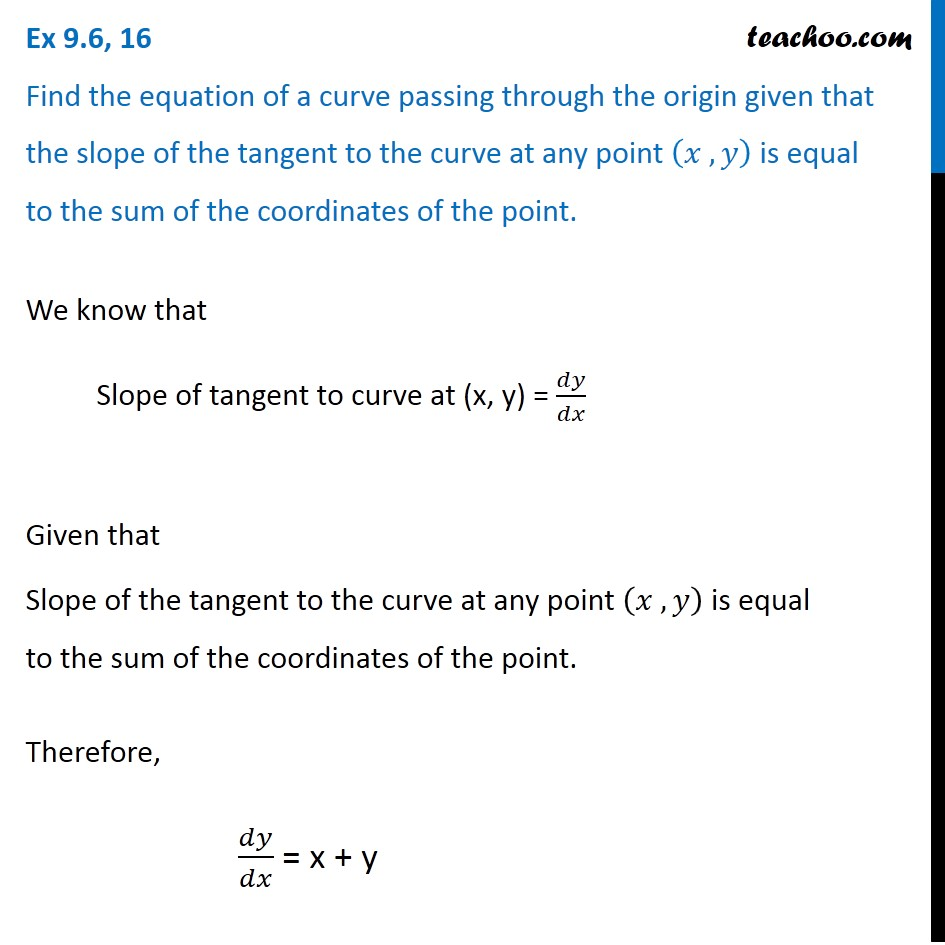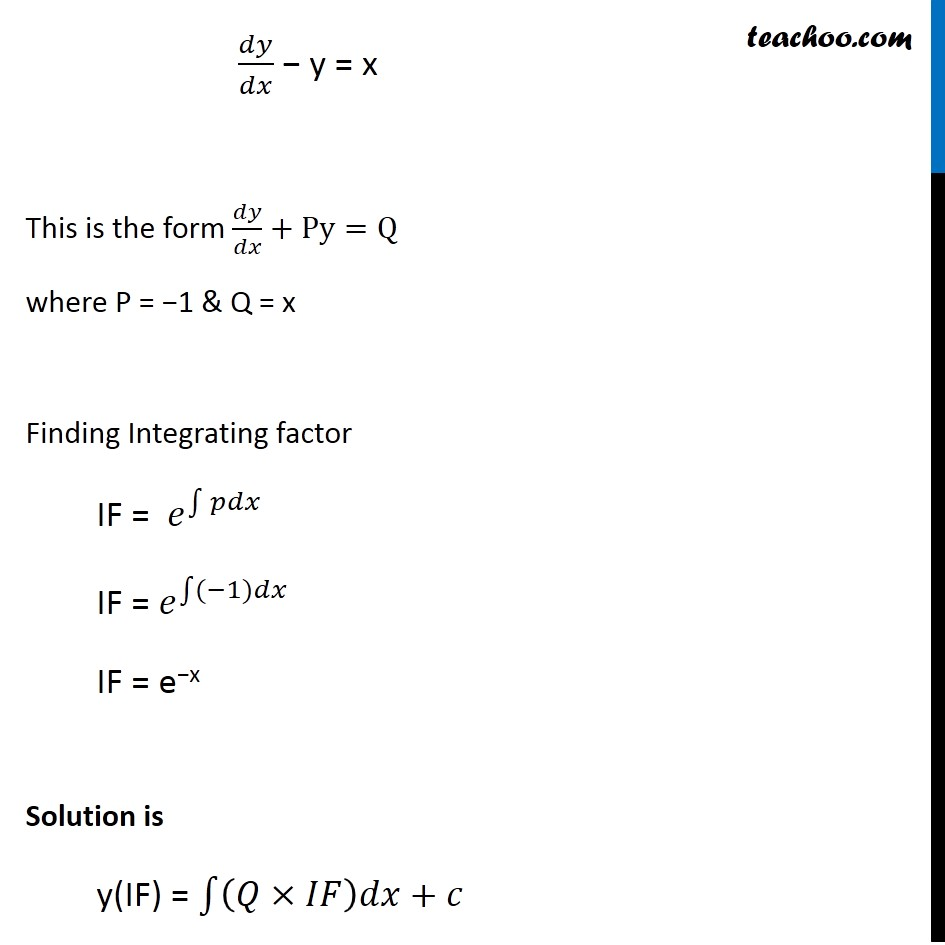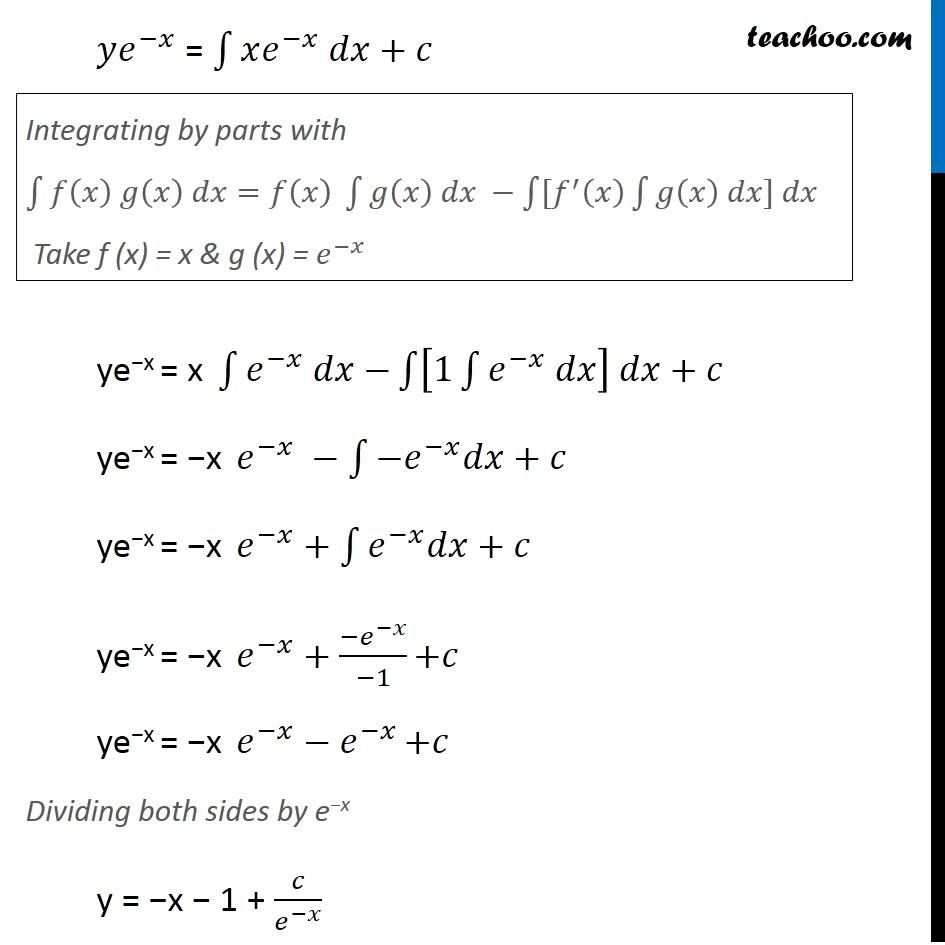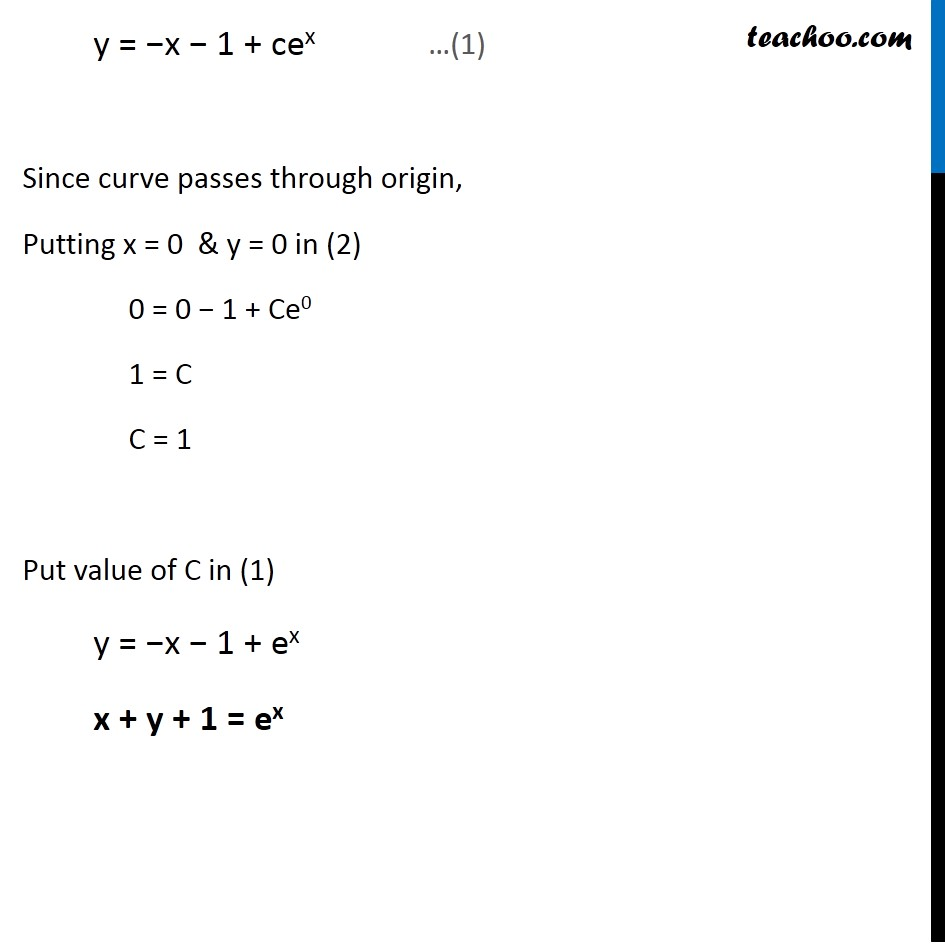Ex 9.5

Chapter 9 Class 12 Differential Equations
Serial order wiseLearn in your speed, with individual attention - Teachoo Maths 1-on-1 Class

### Transcript

Ex 9.5, 16 Find the equation of a curve passing through the origin given that the slope of the tangent to the curve at any point (𝑥 , 𝑦) is equal to the sum of the coordinates of the point. We know that Slope of tangent to curve at (x, y) = 𝑑𝑦/𝑑𝑥 Given that Slope of the tangent to the curve at any point (𝑥 , 𝑦) is equal to the sum of the coordinates of the point. Therefore, 𝑑𝑦/𝑑𝑥 = x + y 𝑑𝑦/𝑑𝑥 − y = x This is the form 𝑑𝑦/𝑑𝑥+Py=Q where P = −1 & Q = x Finding Integrating factor IF = 𝑒^∫1▒〖𝑝𝑑𝑥 〗 IF = 𝑒^∫1▒〖(−1)𝑑𝑥 〗 IF = e−x Solution is y(IF) = ∫1▒〖(𝑄×𝐼𝐹)𝑑𝑥+𝑐〗 𝑦𝑒^(−𝑥) = ∫1▒〖𝑥𝑒^(−𝑥) 𝑑𝑥+𝑐〗 ye−x = x ∫1▒〖𝑒^(−𝑥) 𝑑𝑥−〗 ∫1▒〖[1∫1▒〖𝑒^(−𝑥) 𝑑𝑥〗] 𝑑𝑥+𝑐〗 ye−x = −x 𝑒^(−𝑥) −∫1▒〖−𝑒^(−𝑥) 𝑑𝑥+𝑐〗 ye−x = −x 𝑒^(−𝑥)+∫1▒〖𝑒^(−𝑥) 𝑑𝑥+𝑐〗 ye−x = −x 𝑒^(−𝑥)+(−𝑒^(−𝑥))/(−1) +𝑐 ye−x = −x 𝑒^(−𝑥)−𝑒^(−𝑥) +𝑐 Dividing both sides by e−x y = −x − 1 + 𝑐/𝑒^(−𝑥) Integrating by parts with ∫1▒〖𝑓(𝑥) 𝑔(𝑥) 𝑑𝑥=𝑓(𝑥) ∫1▒〖𝑔(𝑥) 𝑑𝑥 −∫1▒〖[𝑓^′ (𝑥) ∫1▒〖𝑔(𝑥) 𝑑𝑥] 𝑑𝑥〗〗〗〗 Take f (x) = x & g (x) = 𝑒^(−𝑥) y = −x − 1 + cex Since curve passes through origin, Putting x = 0 & y = 0 in (2) 0 = 0 − 1 + Ce0 1 = C C = 1 Put value of C in (1) y = −x − 1 + ex x + y + 1 = ex …(1)